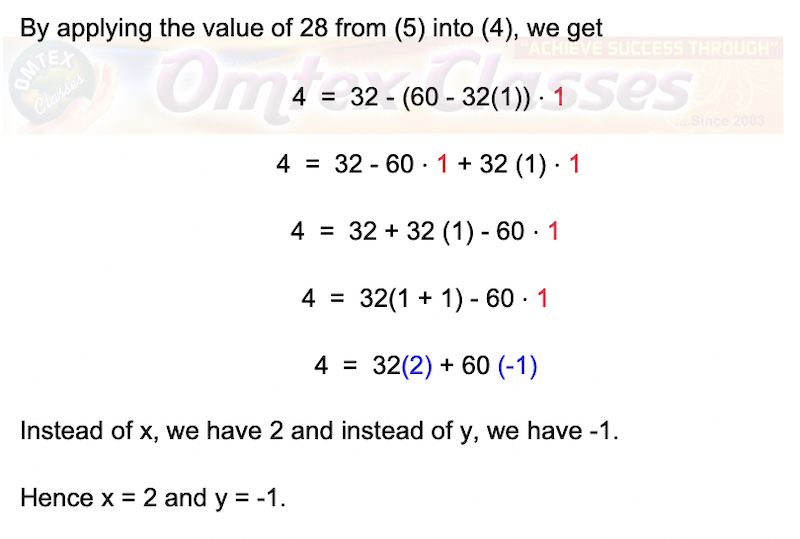### If d is the Highest Common Factor of 32 and 60, find x and y satisfying d = 32x + 60y.

SSLC 10TH STANDARD Tamil Nadu NEW SYLLABUS. 2019 - 2020.

CHAPTER 2: NUMBERS AND SEQUENCES

Exercise: 2.1

Question 8 :

## If d is the Highest Common Factor of 32 and 60, find x and y satisfying d = 32x + 60y.## If d is the Highest Common Factor of 32 and 60, find x and y satisfying d = 32x + 60y.